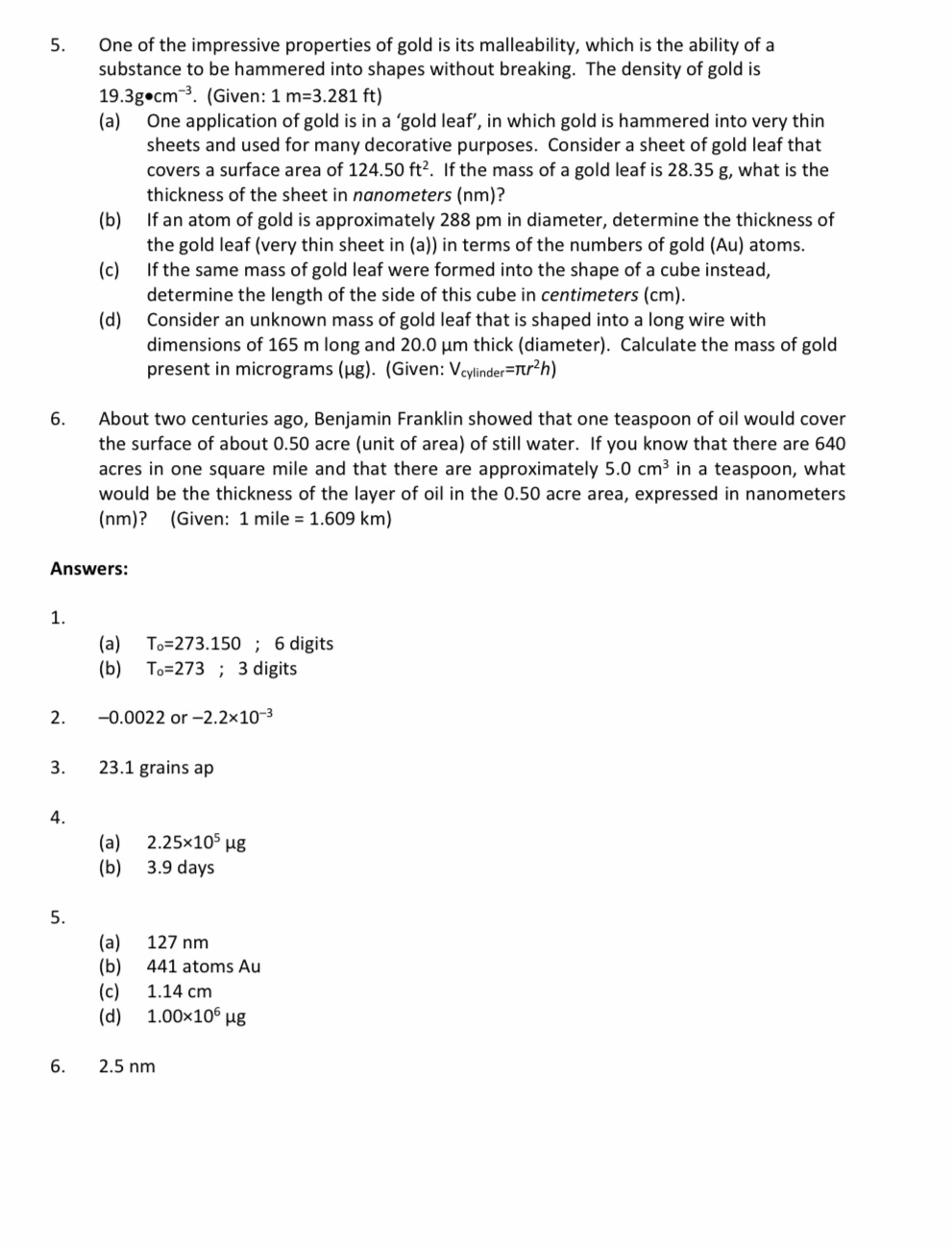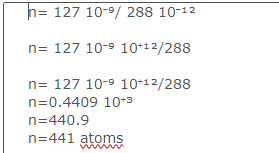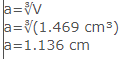# Help Zone

### Student Question

Hi there!

I need explanation for question 5 and 6 as soon as possible because tomorrow is my exam and I’m not able to understand how to answer them. If someone could kindly explain me in details in steps.

The answers are below the questions but I don’t get how to solve them?

thank u so much!Chemistry## Explanations (2)

•Explanation from AlloprofExplanation from Alloprof

This Explanation was submitted by a member of the Alloprof team.

Hi,

Also, we can't look at the whole problem, so you'll have to show us your procedure.

Thank you very much. See you soon :)

• Options
3mo. edited September 2

a)

Density of gold d=19.3 g cm-³

Area of the sheet S=124.5 ft²

h thickness of the sheet to be determined

m mass of gold used m=28.35 g

we have the formula

mass= density x Volume

Volume = Area x thickness

m=dxSxh h=m/d x S

units given

m : g

d : g cm-³

S: ft²

we have to change S in cm²

1 ft = 12 inch

1 inch =2.54 cm

we obtain

1 ft = 12 x 25.4 cm 1ft=30.48 cm

1ft²= 30.48² cm²

1ft²= 929.0304 cm²

S=(124.5 x 929.0305) cm²

h= 28.35 g/(19.3 g cm-³ (124.5 x 929.0305) cm²)

h=28.35 g/(19.3 g cm-³ x 124.5 x 929.0305 cm²)

h=(28.35 /(19.3 x 124.5 x 929.0305 )) (g/g cm-³ cm²)

h=(0.000012699) cm

1 cm =0.01 m

h=(0.000012699) x 0.01 m

h=0.00000012699 m

h=126.99 nm =127 nm

b)

atom gold diameter =288 pm

thickness of the sheet=(number of atoms) x (atom gold diameter)

other wise

h=n*Diameter

n=h/Diameterc)

m = V x d

mass = Volume x density

28.35 g = V x 19.3 g cm-³

V=28.35 g/19.3 g cm-³

V=(28.35/19.3) g/g cm-³

V=1.469 cm³

we consider this volume as a cube

V=a³